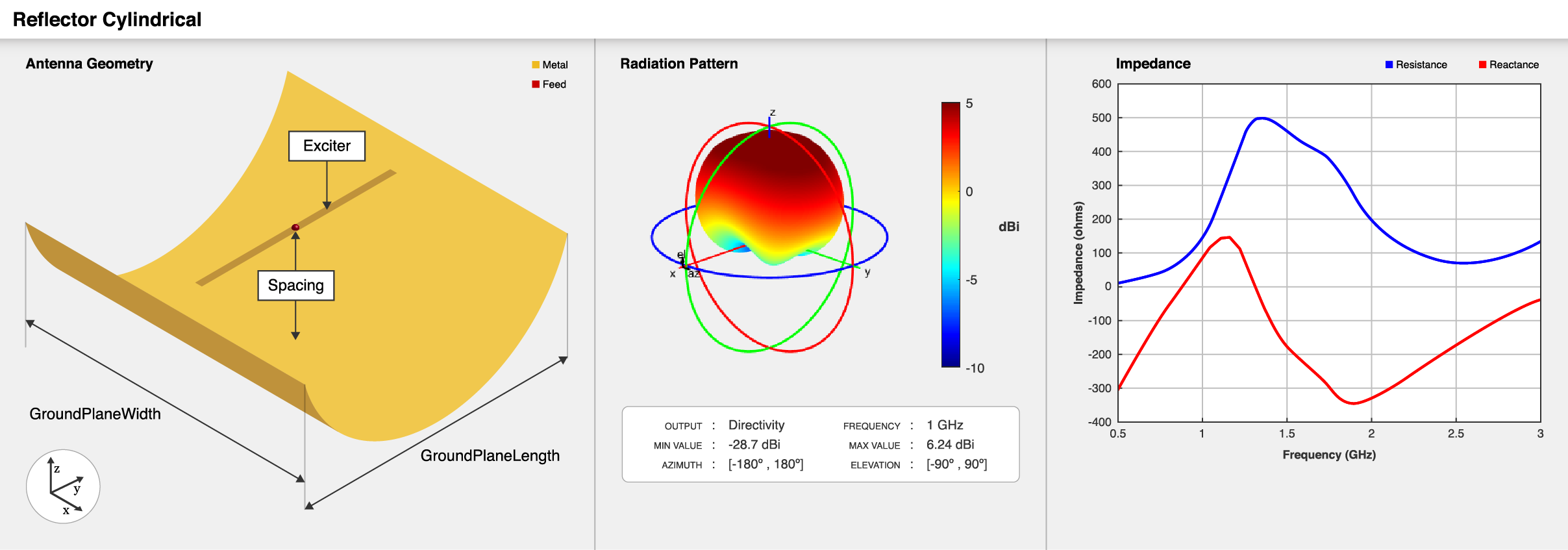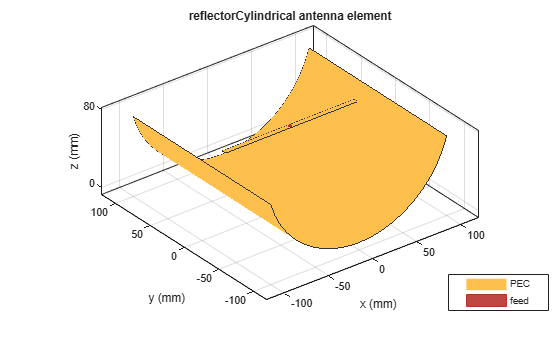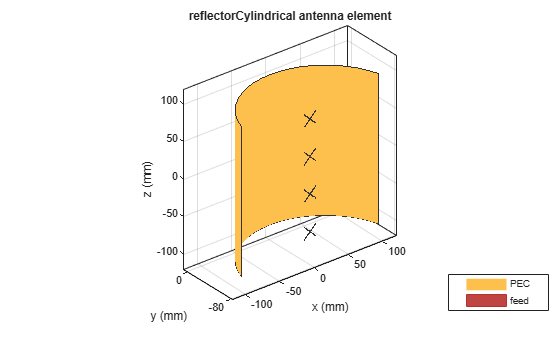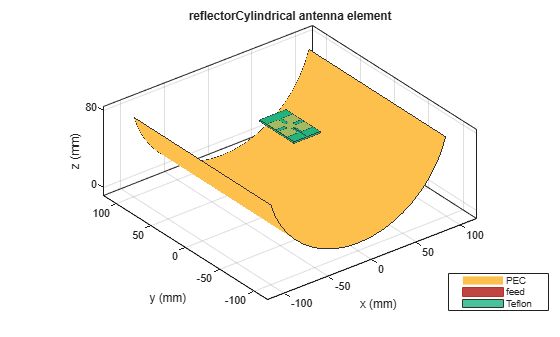# reflectorCylindrical

Create cylindrical reflector-backed antenna

Since R2020b

## Description

The `reflectorCylindrical` antenna object creates a cylindrical reflector-backed antenna. The cylindrical shape of the reflector allows you to focus the signal to the antenna surface. Cylindrical reflectors are widely used as high-gain apertures fed with line sources and in airborne navigational antennas where sharp azimuthal beams and wide-angle vertical coverage is required.## Creation

### Syntax

``ant = reflectorCylindrical``
``ant = reflectorCylindrical(Name=Value)``

### Description

example

````ant = reflectorCylindrical` creates a cylindrical reflector-backed antenna. The default cylindrical reflector antenna object has an exciter as a center-fed dipole located on the X-Y plane and the dimensions are chosen for an operating frequency of around 1 GHz.```

example

````ant = reflectorCylindrical(Name=Value)` creates a cylindrical reflector antenna, with additional Properties specified by one or more name–value arguments. `Name` is the property name and `Value` is the corresponding value. You can specify several name-value arguments in any order as `Name1`= `Value1`, `...`, `NameN`=`ValueN`. Properties not specified retain their default values.For example, `reflectorCylindrical(GroundPlaneWidth=0.21)` creates a cylindrical reflector with a width of 0.21 meters.```

## Properties

expand all

Exciter antenna or array type, specified as an antenna object, an array object, or an empty array. Except for reflector and cavity antenna elements, you can use any Antenna Toolbox™ antenna or array element as an exciter. To create the reflector backing structure without the exciter, specify this property as an empty array.

Example: `dipole`

Example: `linearArray(Element=patchMicrostrip)`

Example: `[]`

Reflector length along X-axis, specified as a positive scalar in meters.

Example: `0.6`

Data Types: `double`

Reflector width along Y-axis, specified as a positive scalar in meters.

Example: `0.4`

Data Types: `double`

Distance between reflector and exciter, specified as a scalar in meters.

Example: `0.059`

Data Types: `double`

Perpendicular distance between the ground plane and the aperture of the cylindrical reflector, specified as a positive scalar in meters.

Example: `0.09`

Note

`Depth` should be less than or equal to half of the GroundPlaneLength.

Data Types: `double`

Flag to enable the probe feed, specified as `0` or `1`. Setting the flag to `0` disables the probe feed, and setting the flag to `1` enables it.

Example: `1`

Data Types: `double`

Type of the metal used as a conductor, specified as a metal material object. You can choose any metal from the `MetalCatalog` or specify a metal of your choice. For more information, see `metal`. For more information on metal conductor meshing, see Meshing.

Example: `metal("Copper")`

Tilt angle of the antenna in degrees, specified as a scalar or vector. For more information, see Rotate Antennas and Arrays.

Example: `90`

Example: `Tilt=[90 90]`,`TiltAxis=[0 1 0;0 1 1]` tilts the antenna at 90 degrees about the two axes defined by the vectors.

Data Types: `double`

Tilt axis of the antenna, specified as one of these values:

• Three-element vector of Cartesian coordinates in meters. In this case, each coordinate in the vector starts at the origin and lies along the specified points on the x-, y-, and z-axes.

• Two points in space, specified as a 2-by-3 matrix corresponding to two three-element vectors of Cartesian coordinates. In this case, the antenna rotates around the line joining the two points.

• `"x"`, `"y"`, or `"z"` to describe a rotation about the x-, y-, or z-axis, respectively.

Example: `[0 1 0]`

Example: `[0 0 0;0 1 0]`

Example: `"Z"`

Data Types: `double` | `string`

Lumped elements added to the antenna feed, specified as a `lumpedElement` object. You can add a load anywhere on the surface of the antenna. By default, the load is at the feed. For more information, see `lumpedElement`.

Example: `Load=lumpedelements`, where `lumpedelements` is the load added to the antenna feed.

Example: `lumpedElement(Impedance=75)`

## Object Functions

 `show` Display antenna, array structures, shapes, or platform `impedance` Input impedance of antenna or scan impedance of array `sparameters` Calculate S-parameters for antennas and antenna arrays `returnLoss` Return loss of antenna or scan return loss of array `vswr` Voltage standing wave ratio (VSWR) of antenna or array element `optimize` Optimize antenna or array using SADEA optimizer `pattern` Plot radiation pattern and phase of antenna or array or embedded pattern of antenna element in array `patternAzimuth` Azimuth plane radiation pattern of antenna or array `patternElevation` Elevation plane radiation pattern of antenna or array `axialRatio` Axial ratio of antenna `beamwidth` Beamwidth of antenna `current` Current distribution on antenna or array surface `charge` Charge distribution on antenna or array surface `efficiency` Radiation efficiency of antenna `EHfields` Electric and magnetic fields of antennas or embedded electric and magnetic fields of antenna element in arrays `mesh` Mesh properties of metal, dielectric antenna, or array structure `design` Design prototype antenna or arrays for resonance around specified frequency or create AI-based antenna from antenna catalog objects `rcs` Calculate and plot radar cross section (RCS) of platform, antenna, or array

## Examples

collapse all

Create a cylindrical reflector antenna object with default properties.

`ant = reflectorCylindrical;`

View the antenna object.

`show(ant)`Create a `reflectorCylindrical` antenna object with a rounded bow-tie dipole antenna as an exciter.

```b = bowtieRounded(Length=96e-3,Tilt=90,TiltAxis=[0 1 0]); r = reflectorCylindrical(Exciter=b,Spacing=100e-3);```

View the antenna object.

```figure show(r)```Plot the radiation pattern at 1.5 GHz.

```figure pattern(r,1.5e9)```Enable the probe feed for the `reflectorCylindrical` antenna object.

`re = reflectorCylindrical(Exciter=b,Spacing=100e-3,EnableProbeFeed=1);`

View the antenna object with the probe feed enabled.

```figure show(re)```Plot the radiation pattern of the antenna object at 1.5 GHz with the probe feed enabled.

```figure pattern(re,1.5e9)```Create a linear array of crossed dipole antenna.

```d = dipoleCrossed(Tilt=90,TiltAxis=[0 1 0]); la = linearArray(Element=d,NumElements=4,ElementSpacing=0.05,Tilt=90,TiltAxis=[0 0 1]);```

Create a cylindrical reflector backed array.

`ant = reflectorCylindrical(Exciter=la,Tilt=90)`
```ant = reflectorCylindrical with properties: Exciter: [1x1 linearArray] GroundPlaneLength: 0.2000 GroundPlaneWidth: 0.2000 Spacing: 0.0750 Depth: 0.0750 EnableProbeFeed: 0 Conductor: [1x1 metal] Tilt: 90 TiltAxis: [1 0 0] Load: [1x1 lumpedElement] ```
`show(ant)`Create a cylindrical reflector-backed Minkowski's loop fractal antenna.

```e = fractalIsland(Substrate=dielectric("Teflon"),Tilt=90,TiltAxis=[0 0 1]); ant = reflectorCylindrical(Exciter=e)```
```ant = reflectorCylindrical with properties: Exciter: [1x1 fractalIsland] GroundPlaneLength: 0.2000 GroundPlaneWidth: 0.2000 Spacing: 0.0750 Depth: 0.0750 EnableProbeFeed: 0 Conductor: [1x1 metal] Tilt: 0 TiltAxis: [1 0 0] Load: [1x1 lumpedElement] ```
`show(ant)`Balanis, Constantine A. Antenna Theory: Analysis and Design. 3rd ed. Hoboken, NJ: John Wiley, 2005.